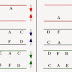Seating arrangement is one of the most important question of General awareness and Reasoning section of SSC CGL Tier-I. In almost every exam, one set from this topic always appear. For these types of questions, you just need to read the information carefully first, then make a mindmap and start solving questions.

### Examples with Solution

Example1: Read the following information’s carefully and answer the questions given below it:
• Six friends A, B, C, D, E and F are standing in two rows facing one another.
• A is in the middle of one row.
• F is the left of D and facing C.
• B and E are not in the same row.
• Only one person between C and E.

Ques1: Which of the following in the same Row?
A. F, D, and E
B. F, B, and A
C. C, A and E
D. F, C and B

Ques2: After interchanging the places of C and E who is the right of E?
A. A
B. C
C. F
D. None of these

Ques3: Who is the left of D?
A. A
B. F
C. D
D. B

Ques4: Who faces A?
A. B
B. E
C. D
D. F

Ques5: Which of the following pairs are NOT facing each other?
A. CD
B. EF
C. AF
D. EB

Ques6: Who among the following are facing each other?
A. AB
B. CF
D. EB

Ques7: Which of the following statements is definitely true?
A. AB is facing each other
B. E faces A
C. F is the left of D
D. None of these

Solution: For these types of questions, firstly You need to make arrangements according to statements given in question.

See the following arrangements, statement wise. There can be two types of arrangements. You can use any of these.First arrangement (in red) shows A is in the middle of one row.

Second arrangement (in blue) shows F is the left of D and facing C.

Third arrangement i.e. final arrangement (in green) shows B and E are not in the same row.
Only one person between C and E.

1. Out of given options, C,A,E are in same row.

2. Interchanging positions of C and E, right of E will be 'A'.

3. Left of D = 'F'

4. Faces A = 'F'

5. Out of given options 'EF' not facing each other.

6. Out of given options, 'EB' are facing each other.

7. Ab is not facing each other. Therefore, first option is false.
E is not facing A, second option also wrong
F is left of D, therefore, 3rd option is right.

See it becomes very easy to answer all the questions in a set, if you are able to make the arrangements in a right way. If out of all the questions, any one of question is giving you a confusing answer, that means you did wrong arrangements. So, firstly try to make a mind map or the arrangements accurately. I'm providing you a question for practice. Try to do it yourself. For any query or problem, you are free to contact me.

### Problem for Practice

Problem: Read the following information carefully and answer the questions given below:
• A, B, C, D, E and F are six friends are sitting in a circle facing the center.
• F is the 4th left of E.
• A is not between D and E but some other one.
• C is next left of F.
Questions:

1. Who is left of D?
2. Who is right of E?
3. Who is third right of C?
4. Who is facing D?
5. If F and E interchanging the positions then which of the following pair will sit together?
6. What is the position of E?
7.  Who is sitting just right to D?
Inform me if anyone need its solution. Thanks for visiting.
Seating Arrangement Examples for SSC CGL Tier-IReviewed by Jasleen Behl on Tuesday, February 18, 2014 Rating: 5

1.The Solved Example is its given as F is the left of D facing C. Should it not be D is facing C? Otherwise the statement will make F facing C

And pls check whether my answers for unsolved is right or wrong for the second sum
1. B
2. A
3. E
4. A
7. B

2.sol plz

I will try to respond asap